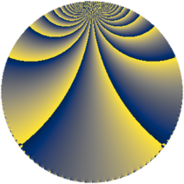Properties

 Label 684.3.mLevel $684$ Weight $3$ Character orbit 684.m Rep. character $\chi_{684}(353,\cdot)$ Character field $\Q(\zeta_{6})$ Dimension $80$ Newform subspaces $1$ Sturm bound $360$ Trace bound $0$

Related objects

Defining parameters

 Level: $$N$$ $$=$$ $$684 = 2^{2} \cdot 3^{2} \cdot 19$$ Weight: $$k$$ $$=$$ $$3$$ Character orbit: $$[\chi]$$ $$=$$ 684.m (of order $$6$$ and degree $$2$$) Character conductor: $$\operatorname{cond}(\chi)$$ $$=$$ $$171$$ Character field: $$\Q(\zeta_{6})$$ Newform subspaces: $$1$$ Sturm bound: $$360$$ Trace bound: $$0$$

Dimensions

The following table gives the dimensions of various subspaces of $$M_{3}(684, [\chi])$$.

Total New Old
Modular forms 492 80 412
Cusp forms 468 80 388
Eisenstein series 24 0 24

Trace form

 $$80 q - 2 q^{3} + q^{7} - 2 q^{9} + O(q^{10})$$ $$80 q - 2 q^{3} + q^{7} - 2 q^{9} + 18 q^{11} - 5 q^{13} - 2 q^{15} - 9 q^{17} + 20 q^{19} - 30 q^{21} + 72 q^{23} - 400 q^{25} + 25 q^{27} - 8 q^{31} - 64 q^{33} + 22 q^{37} + 39 q^{39} - 44 q^{43} - 196 q^{45} - 267 q^{49} - 47 q^{51} - 36 q^{53} + 84 q^{57} - 14 q^{61} - 260 q^{63} - 144 q^{65} - 77 q^{67} + 44 q^{69} - 135 q^{71} + 43 q^{73} + 69 q^{75} + 216 q^{77} - 17 q^{79} - 254 q^{81} - 171 q^{83} - 244 q^{87} + 216 q^{89} + 122 q^{91} + 292 q^{93} - 288 q^{95} - 8 q^{97} + 172 q^{99} + O(q^{100})$$

Decomposition of $$S_{3}^{\mathrm{new}}(684, [\chi])$$ into newform subspaces

Label Dim. $$A$$ Field CM Traces $q$-expansion
$a_{2}$ $a_{3}$ $a_{5}$ $a_{7}$
684.3.m.a $80$ $18.638$ None $$0$$ $$-2$$ $$0$$ $$1$$

Decomposition of $$S_{3}^{\mathrm{old}}(684, [\chi])$$ into lower level spaces

$$S_{3}^{\mathrm{old}}(684, [\chi]) \cong$$ $$S_{3}^{\mathrm{new}}(171, [\chi])$$$$^{\oplus 3}$$$$\oplus$$$$S_{3}^{\mathrm{new}}(342, [\chi])$$$$^{\oplus 2}$$